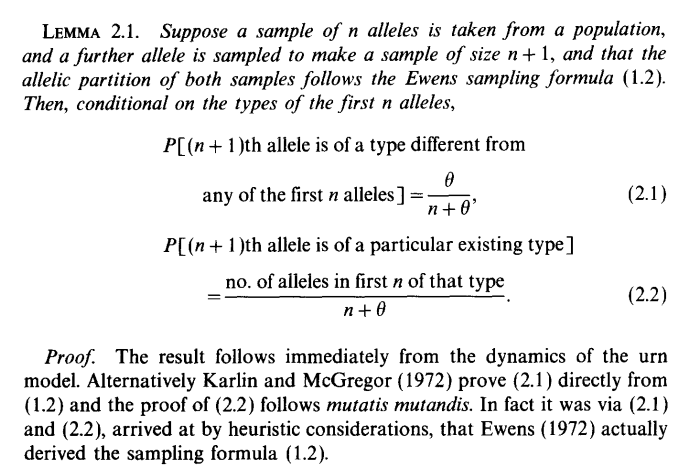On page 289 onwards, Hubbell (2001) gives an algorithm for sequentially sampling individuals from the neutral metacommunity and obtaining their species identities. The algorithm makes use of a quantity he calls the ‘species generator’

$\frac{\theta}{\theta + j - 1}$

where $$\theta$$ is the “fundamental biodiversity number” and $$j$$ is the index of the individual drawn (1st, 2nd, …). In Figure 9.1 he gives a flow diagram for the algorithm, but it is perhaps easier to understand from reading code directly.

From the hubbell package for R, Jari Oksanen authored this code:

Unfortunately, Hubbell (2001) did not cite a paper for this method, instead attributing Warren Ewens with a pers. comm. However, I found the equivalent expression in Donnelly (1986), Lemma 2.1.Etienne (2005) provides some historical context:

Distributions (sampling formulas) in the Ewens family correspond to sequential construction schemes, also called urn schemes, that generate samples from the distribution… These urns are known as Hoppe urns in population genetics, after Hoppe (1984, 1987).

Donnelly, P. (1986). Partition structures, Polya urns, the Ewens sampling formula, and the ages of alleles, Theoretical Population Biology, 30(2): 271-288.

Etienne, R.S. (2005). A new sampling formula for neutral biodiversity. Ecology letters, 8(3): 253-260.

Hubbell, S. P. (2001). The unified neutral theory of biodiversity and biogeography, Princeton University Press, Princeton, USA.